4WX Internet Search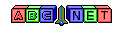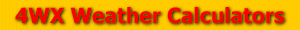# Speed of Sound

From the user, an air temperature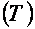is given. Before calculating the speed of sound, the air temperature value must be converted to Kelvin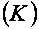.

To convert the air temperature to Kelvin, see the link below:

Just a side note before calculating the speed of sound, the speed of sound is dependent on the density of the air. The density of the air is dependent on the temperature of the air.

Then, the speed of sound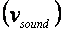can be calculated using the formula below:Back to 4WX Weather Calculators index page

4WX Calculators Credit and disclaimer